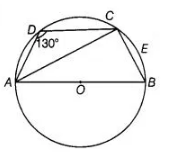# A quadrilateral ABCD is inscribed in a circle`
Question:

A quadrilateral ABCD is inscribed in a circle such that AB is a diameter and ∠ADC = 130°. Find ∠BAC.

Solution:

Draw a quadrilateral $A B C D$ inscribed in a circle having centre $O$. Given, $\angle A D C=130^{\circ}$

Since, $A B C D$ is a quadrilateral inscribed in a circle, therefore $A B C D$ becomes a cyclic quadrilateral.$\because$ Since, the sum of opposite angles of a cyclic quadrilateral

is $180^{\circ}$,

$\therefore$ $\angle A D C+\angle A B C=180^{\circ}$

$\Rightarrow$ $130^{\circ}+\angle A B C=180^{\circ}$

$\Rightarrow$ $\angle A B C=50^{\circ}$

Since, $A B$ is a diameter of a circle, then $A B$ subtends an angle to the circle is right angle.

$\therefore$ $\angle A C B=90^{\circ}$

In $\triangle A B C$, $\angle B A C+\angle A C B+\angle A B C=180^{\circ} \quad$ [by angle sum property of a triangle]

$\Rightarrow \quad \angle B A C+90^{\circ}+50^{\circ}=180^{\circ}$

$\Rightarrow$ $\angle B A C=180^{\circ}-\left(90^{\circ}+50^{\circ}\right)$

$=180^{\circ}-140^{\circ}=40^{\circ}$